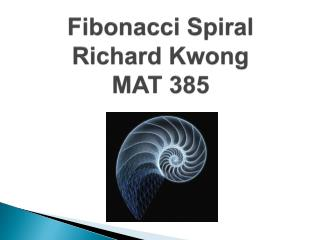DownloadDownload PresentationFibonacci Spiral Richard Kwong MAT 385

# Fibonacci Spiral Richard Kwong MAT 385

Télécharger la présentation## Fibonacci Spiral Richard Kwong MAT 385

- - - - - - - - - - - - - - - - - - - - - - - - - - - E N D - - - - - - - - - - - - - - - - - - - - - - - - - - -
##### Presentation Transcript

1. Fibonacci SpiralRichard KwongMAT 385

2. What is the Fibonacci Spiral? • The Fibonacci Spiral is created from the Fibonacci Sequence. The Fibonacci sequence is a mathematical succession of numbers in which each number is obtained by adding together the last 2 digits that came before it. • The sequence goes as follows: 0, 1, 1, 2, 3, 5, 8, 13, 21, 34, 55, 89, 144, 233..

3. Fibonacci Sequence in Nature • The Fibonacci series is present all around us in many different forms such as flower patterns and shells. One of the best representations is in the sunflower. The pattern of seeds within a sunflower follows the Fibonacci sequence.

4. Fibonacci Sequence in Nature cont. • Another well known representation of the Fibonacci sequence is found in pinecones. In In the picture, there are 8 spirals bending to the left as they open out indicated in red.Likewise there are 13 spirals opening to the right indicated by blue traces.8 and 13 are consecutive Fibonacci numbers.

5. Step 1 Begin by drawing a square inside the Rectangle. The length of each side is equal to the height of the frame.

6. Step 2 Draw an arc within the square you just drew with a radius that is equal to the height of the frame.

7. Step 3 Draw next square with sides that are equal to the smallest length of the rectangle on the right. The arc is then drawn into the square as well.

8. Step 4 Continue iteration..

9. Step 5 The Fibonacci Spiral is now finished being drawn.